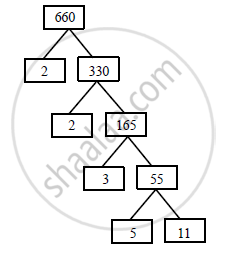# Find the Missing Numbers in the Following Factorization: - Mathematics

Find the missing numbers in the following factorization:

#### Solution

660 = 2 × 2 × 3 × 5 × 11Concept: Euclid’s Division Lemma
Is there an error in this question or solution?